# Calculating the Average for Numbers Meeting Specified Criteria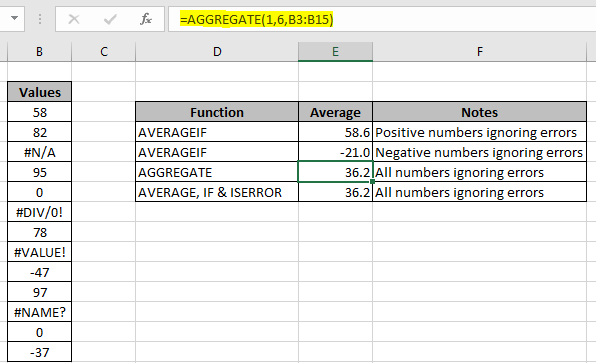In this article, we will learn Calculating the Average for Numbers Meeting Specified Criteria in Excel.

Scenario:

When working with data, where we need to get the average of numbers having criteria values. Why criteria values create a difference in result, excel function should consider it zero and take the result. A zero value can make a difference in Average of numbers. Average of numbers is the sum of required numbers divided by count of numbers. Where the zero doesn't contribute to the sum but it does increase the count. Understand how to solve the problem.

How to solve the problem?

Here we need to find the average of numbers with criteria. Criteria is ignoring criteria values. If you have only positive numbers or negative numbers you can use AVERAGEIF function

Formula syntax (positive numbers):

 =AVERAGEIF(values, ">=0")

The above formula includes zeroes but ignores criteria values. If you want to ignore zeros and criteria values, then just use the criteria as ">0".

Similarly you can get the average of negative values including zeros using the formula:

Formula syntax (negative numbers):

 =AVERAGEIF(values, "<=0")

The above formula includes zeroes but ignores criteria values. If you want to ignore zeros and criteria values, then just use the criteria as ">0"

But the problem of considering numbers ignoring criterias is not solved. You might think that, taking the average of the above two results (average of positive numbers and average of negative numbers) will give the required value. It doesn't work well until the count of positive numbers equals the count of negative numbers. For this problem we will use the AGGREGATE function. AGGREGATE function is the package of mathematical functions. It helps in generally solving these kinds of problems. To unpack this package for the average of values ignoring criterias. Use the below formula.

Formula syntax (for all numbers):

 =AGGREGATE(1, 6, values)

1 : Argument to Average numbers

6 : ignoring criteria values

Example :

All of these might be confusing to understand. Let's take an example and understand how to use these formulas in an example. Here we have a list of values for which average is required.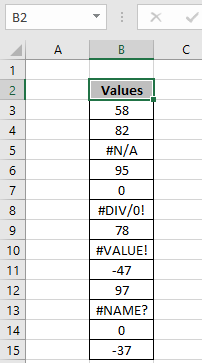Firstly we calculate the average for the positive numbers. For this we will use the below for the data.

Use the formula:

 =AVERAGEIF(B3:B15,">=0")

Explanation

1. Criteria is numbers which are greater than or equal to zero.
2. AVERAGEIF function considers only the numbers which satisfy the criteria and returns the average of the numbers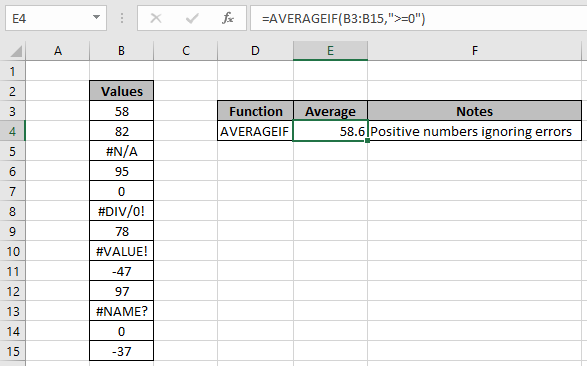Here the formula returns 58.6 considering only the positive values where count of positive values is 7. Similarly you can get the average of the negative numbers.

Use the formula :

 =AVERAGEIF(B3:B15,"<=0")

Explanation

1. Criteria is numbers which are less than or equal to zero.
2. AVERAGEIF function considers only the numbers which satisfy the criteria and returns the average of the numbers.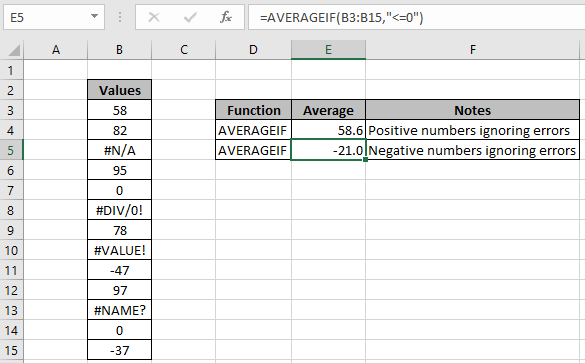For negative numbers the average is -21.0 including zeros ignoring criteria values. Count to considered numbers is 4.

Here in this example the count of negative numbers is not equal to the count of positive numbers. So the average of the 2 above results will not yield the average of all numbers. Use the below formula for these cases.

Use the formula :

 =AGGREGATE(1, 6, B3:B15)

Explanation:

1. Argument 1 is given to use Average function.
2. Argument 6 is given to ignore criteria values.
3. AGGREGATE function returns the average of all numbers in B3:B15 array ignoring criteria values.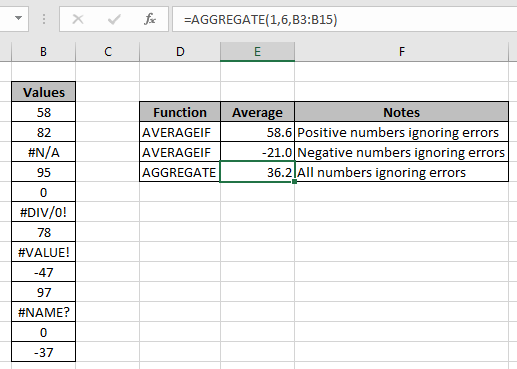The average of all numbers ignoring all criteria values comes out to be 36.2. Here the count of numbers is 9. AGGREGATE function is the best way to solve these problems, as these functions are excel inbuilt functions.

If you are trying to get the average of numbers using the basic AVERAGE function, you need to use the below formula to get the average of numbers ignoring criterias.

Use the formula :

 { =AVERAGE(IF(IScriteria(B3:B15),"",B3:B15)) }

Note : do not use the curly braces manually. Just use Ctrl + Shift + Enter in place of Enter to get the result.

Explanation:

1. IF function checks the condition with IScriteria function on the B3:B15 array.
2. IScriteria function returns True for the value where value is an criteria and False where not.
3. IF function returns blank value if value is criteria and returns the corresponding value if value is not criteria.
4. AVERAGE function returns the average of the returned values.
5. Curly braces make these logical functions work for an array.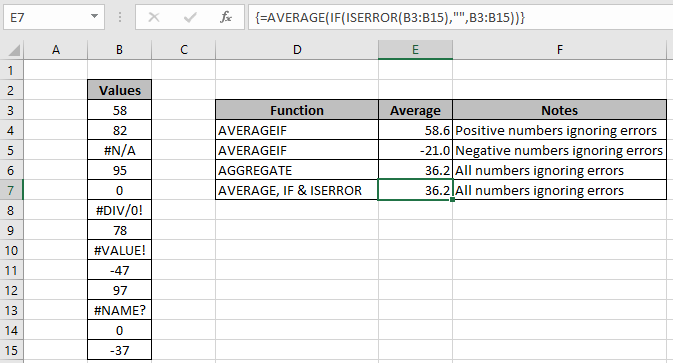As you can see the formula works fine and returns the same result. But use the AGGREGATE function, it's easy to use for various mathematical functions with different inbuilt criteria.

If you don't have any zero value in the array. You can use the return zero out of all the criteria values and use the formula how to take the average of numbers ignoring zeros here. But this formula doesn't work with criteria values.

Here are all the observational notes regarding using the formula.

Notes:

1. AGGREGATE function is the best function to use for these problems.
2. The function returns #VALUE! criteria if no value matches the given criteria.
3. Different Criteria inside formula is accepted, such as equals to (=), less than (<) , less than or equals to (<=) , greater than or equals to (>=) , greater than (>) & not equals to (<>).

In this way, we learnt Calculating the Average for Numbers Meeting Specified Criteria in Excel. You can use these functions in Excel 2016, 2013 and 2010. Find more articles on Mathematical formulation with different criteria. If you liked our blogs, share it with your friends on Facebook. And also you can follow us on Twitter and Facebook. We would love to hear from you, do let us know how we can improve, complement or innovate our work and make it better for you. Write us at info@exceltip.com

Related Articles

How To Highlight Cells Above and Below Average Value : highlight values which are above or below the average value using the conditional formatting in Excel.

Ignore zero in the Average of numbers : calculate the average of numbers in the array ignoring zeros using AVERAGEIF function in Excel.

Calculate Weighted Average : find the average of values having different weight using SUMPRODUCT function in Excel.

Average Difference between lists : calculate the difference in average of two different lists. Learn more about how to calculate average using basic mathematical average formula.

Average numbers if not blank in Excel : extract average of values if cell is not blank in excel.

AVERAGE of top 3 scores in a list in excel : Find the average of numbers with criteria as highest 3 numbers from the list in Excel

Popular Articles:

How to use the IF Function in Excel : The IF statement in Excel checks the condition and returns a specific value if the condition is TRUE or returns another specific value if FALSE.

How to use the VLOOKUP Function in Excel : This is one of the most used and popular functions of excel that is used to lookup value from different ranges and sheets.

How to use the COUNTIF Function in Excel : Count values with conditions using this amazing function. You don't need to filter your data to count specific values. Countif function is essential to prepare your dashboard.

How to use the SUMIF Function in Excel : This is another dashboard essential function. This helps you sum up values on specific conditions.

Terms and Conditions of use

The applications/code on this site are distributed as is and without warranties or liability. In no event shall the owner of the copyrights, or the authors of the applications/code be liable for any loss of profit, any problems or any damage resulting from the use or evaluation of the applications/code.